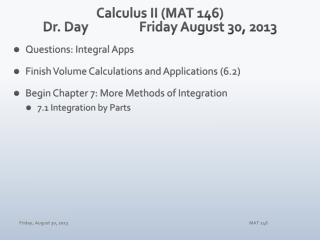# Calculus II (MAT 146) Dr. Day Friday August 30, 2013 - PowerPoint PPT PresentationDownload PresentationCalculus II (MAT 146) Dr. Day Friday August 30, 2013

Calculus II (MAT 146) Dr. Day Friday August 30, 2013Download Presentation## Calculus II (MAT 146) Dr. Day Friday August 30, 2013

- - - - - - - - - - - - - - - - - - - - - - - - - - - E N D - - - - - - - - - - - - - - - - - - - - - - - - - - -
##### Presentation Transcript

1. Calculus II (MAT 146)Dr. Day Friday August 30, 2013 • Questions: Integral Apps • Finish Volume Calculations and Applications (6.2) • Begin Chapter 7: More Methods of Integration • 7.1 Integration by Parts MAT 146

2. Review: Integral Apps (1) Calculate the exact value of the area between the curves f(x) = x3 – x2 – 2x + 1 and g(x) = x2 – 2x + 1. Show calculus and algebraic evidence for all aspects of your solution process. (2) Determine the average value of f(x) = sin(x) on the interval [0,π]. How many values ckexist, on [0,π], such that f(ck) generates that average value? Provide evidence for your responses. MAT 146

3. MAT 146

4. MAT 146

5. MAT 146

6. MAT 146

7. Volumes of Solids of Revolution (6.2 & 6.3) • Dynamic Illustration #1 (discs) • Dynamic Illustration #2 (washer) • Dynamic Illustration #3 (shell) • Dynamic Illustration #4 (cross section I) (cross section II) MAT 146

8. MAT 146

9. Undoing the Product Rule MAT 146

10. Integration by Parts • An Integral with a Product Integrand • Examples MAT 146

11. Integration by Parts • Key Component of Integrand’s Two Factors • For at least one factor, its derivative is “simpler” than the factor. • For at least one factor, its anti-derivative is no more complex than the factor. MAT 146

12. MAT 146

13. You Choosing U: A Decision Algorithm L: log functions I: inverse trig functions A: algebraic functions T: trig functions E: exponential functions MAT 146

14. Assignments WebAssign • 6.2 Homework due Tuesday at midnight • Calc I Review: WA Tasks due Sunday and Tuesday • 7.1 (IBP) Test #1: Thursday/Friday Sept 12-13 MAT 146